# Statistical Hypotheses, Testing of

The following article is from The Great Soviet Encyclopedia (1979). It might be outdated or ideologically biased.

## Statistical Hypotheses, Testing of

a system of procedures in mathematical statistics for the verification of whether experimental data conform to some statistical hypothesis. The use of these procedures permits the acceptance or rejection of statistical hypotheses that arise in the processing or interpretation of measurement results in many areas of science and industry that are of practical importance and involve experiment.

A rule according to which a given hypothesis is accepted or rejected is called a test. A test is defined in terms of a function T of the observation results, which serves as a measure of the discrepancy between the experimental and hypothetical values. This function, which is known as the test statistic, is a random variable. It is assumed here that the probability distribution of T can be calculated when the hypothesis being tested is assumed to be true. On the basis of the distribution of T, a value T0 is chosen such that if the hypothesis is true, the probability of the inequality T > T0 is equal to α, where α is a significance level that is determined in advance. If in actuality it is found that T > T0, then the hypothesis is rejected. On the other hand, the appearance of a value of TT0 does not contradict the hypothesis.

Suppose, for example, the hypothesis must be tested that the independent observation results x1,...,xn are normally distributed with mean a = a0 and known variance σ2. Under this assumption, the arithmetic mean x̄ = (x1 + · · · + xn)/n of the observation results is normally distributed with mean a = a0 and variance σ2/n, and the quantity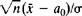is normally distributed with parameters (0, 1). By setting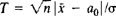, the relationship between T0 and α can be found from normal distribution tables. Under, for example, the hypothesis a = a0, the probability α of T > 1.96 is equal to 0.05. The rule recommending that the hypothesis a = a0 be regarded as false if T > 1.96 will lead to an incorrect rejection of the hypothesis in five cases out of 100 where the hypothesis is true. If, however, T ≤ 1.96, it does not necessarily follow that the hypothesis is confirmed, since the indicated inequality can be satisfied with high probability for a that are close to a0. Consequently, when the proposed test is used, it can be asserted only that the observation results do not contradict the hypothesis a = a0.

In choosing the statistic T, the alternative hypotheses to a = a0 are always taken explicitly or implicitly into account. Suppose, for example, it is known in advance that a ≥ a0—that is, rejection of the hypothesis a = a0 entails acceptance of the hypothesis a > a0. Instead of T there should then be used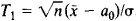If the variance σ2 is unknown, Student’s test can be used instead of the given test for verifying the hypothesis a = a0. Student’s test is based on the statistic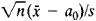which includes an unbiased estimate of the variance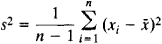and obeys Student’s distribution with n−1 degrees of freedom. (A similar problem is represented in STATISTICS, MATHEMATICAL, Table Ia.) Tests of this kind are known as goodness-of-fit tests and are used in testing hypotheses on the parameters of a distribution and hypotheses on distributions (seeNONPARAMETRIC METHODS).

In using a test based on observation results to decide whether to accept or reject a hypothesis H0, two kinds of errors may be made. An error of the first kind, or Type I error, is committed if H0 is rejected when it is true. An error of the second kind, or Type II error, is committed if H0 is accepted when it is false and some alternative hypothesis H is true. It is natural to require that the test applied to a given hypothesis result in as few erroneous decisions as possible. The usual procedure for obtaining the optimum test for a simple hypothesis is to select from among the tests with a given significance level a, which is the probability of committing an error of the first kind, the test that results in the smallest probability of committing an error of the second kind. In other words, the test is selected that yields the greatest probability of rejecting a hypothesis if it is false. This probability, which is equal to 1 minus the probability of an error of the second kind, is called the power of the test. Where the alternative hypothesis H is simple, the optimum test is the test that is the most powerful of all the other tests with the given level of significance a. If the alternative hypothesis H is composite—for example, if it depends on a parameter—then the power of the test is a function defined on the class of simple alternatives that make up H —that is, the power is a function of the parameter. A test that is simultaneously most powerful against all possible alternatives of the class H is called uniformly most powerful. It should be noted, however, that such a test exists only in a few special situations. A uniformly most powerful test exists in the problem of testing the hypothesis on the mean value of the normal population a = a0 against the alternative hypothesis a > a0, but when the same hypothesis is tested against the alternative aa0, there is no uniformly most powerful test. For this reason, the search for uniformly most powerful tests is often limited to certain special classes, such as invariant or unbiased tests.

The theory of statistical hypothesis testing permits various practical problems of mathematical statistics to be treated from a single point of view. Such problems include the estimation of the difference between mean values, the testing of the hypothesis of constant variance, the testing of the hypothesis of independence, and the testing of hypotheses on distributions. The application of the ideas of sequential analysis to statistical hypothesis testing raises the possibility of linking the decision to accept or reject a hypothesis with the result of sequentially made observations. In this case, the number of observations on the basis of which the decision is made in accordance with a definite rule is not fixed in advance; instead, this number is determined in the course of the experiment. (See also.)

### REFERENCES

Cramer, H. Matematicheskie metody statistiki, 2nd ed. Moscow, 1975. (Translated from English.)
Lehmann, E. Proverka staticheskikh gipotez. Moscow, 1964. (Translated from English.)

A. V. PROKHOROV